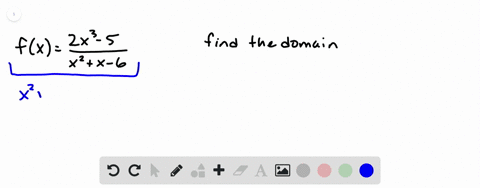Enroll in one of our FREE online STEM summer camps. Space is limited so join now!View Summer Courses## Discussion

You must be signed in to discuss.

## Video Transcript

we want to find the domain of this function and remember that the domain is the set of rial number inputs that would yield rial number outputs. And when you're looking at a rational function like this, you want to focus on the denominator. Remember that division by zero is undefined, and so the denominator cannot be equal to zero. So let's figure out what numbers would make the denominator equal. Zero. If X squared minus nine was equal to zero, then X squared would be equal to nine and X would be equal to plus or minus three. So we're saying that those numbers cannot be in the domain and everything else can. So we have different ways of expressing the domain. One way is to use interval notation so we would have all the numbers from negative infinity to negative three round brackets showing that you're not including it union, Which means or and then all the numbers from negative 3 to 3 again round Brackett, showing that you're not including it and then union, which means or again, and then the interval from three to infinity. So that's one way of saying all real numbers except X equals three and X equals negative three